# How Does Gradient Descent Algorithm Work?

August 6, 2021

lang: en_US

Gradient descent is an optimization approach that determines the values of a function's parameters (coefficients) that minimizes a cost function (cost). This blog post tries to provide you some insight into how optimized gradient descent algorithms behave. We'll start by looking at the many types of gradient descent. Then we'll go over some of the issues encountered during operations. Following that, we'll take a quick look at gradient descent methods and architectures in parallel and distributed scenario.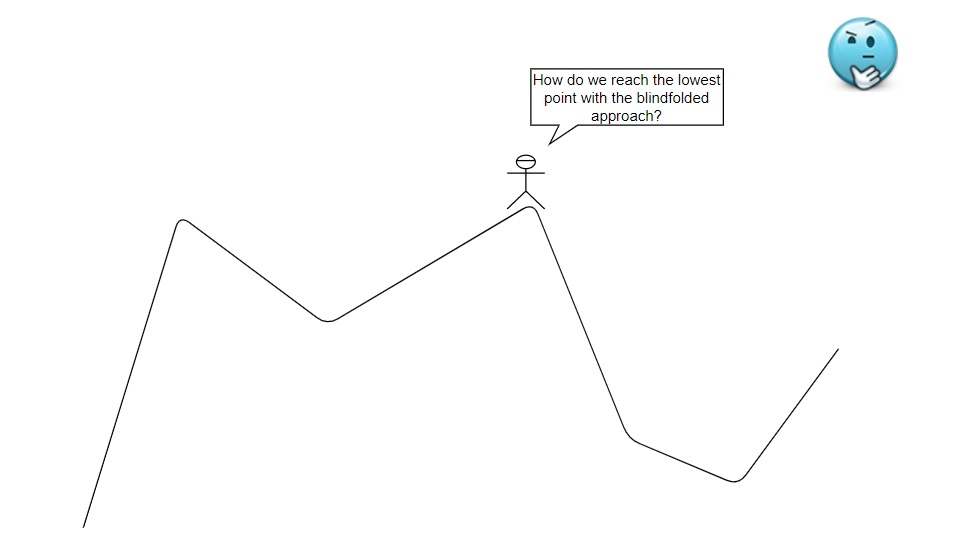The minimum point of the Mountain

Let's say we're at the top of a mountain, and we're given the task of reaching the mountain's lowest point while blindfolded. The most effective way is to look at the ground and see where the landscape slopes down. From there, take another step down until you've reached the lowest point. A similar approach will be taken by the gradient descent method. Gradient descent is an iterative optimization method for locating the function's local minimum. To achieve the stated objectives, it iteratively conducts two phases: The first step is to determine the function's gradient (slope) at that moment, i.e., the first-order derivative. We'll take steps (move) in the opposite direction of the gradient in the next step, raising the slope by alpha times the gradient at that point from where we are now.

Now the question is, what is alpha, and how are we implementing it? At the same time, we can wonder why a minimum point is sometimes referred to as a global minima. If we can identify global minima, we will have an optimum solution to the problem. The optimal solution has a low-cost function. But what is a cost function, how do we achieve an optimum cost function, and how do we use cost function in which circumstances?

## Simple Linear Regression

The purpose of the regression issue is to find the best-fitting line for the data. Let's talk about regression analysis before we get into the actual topic. The table below shows a salary breakdown by age group. The coefficient and intercept values in the following data set are 3 and 4, respectively.

 X (Age in Years) 20 25 30 35 Y (Salary in RS.) 10,000 20,000 Unknown 40,000

We already know what the line equation is.

Equation of the line: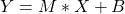;

Now combine the data from the preceding table to produce an unknown value.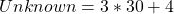This is how we can figure out what a value is that we don't know about. Take the most recent statistics into consideration, and the question now is how to compute the salary of a 32-year-old.

 X (Age in Years) 30 40 50 Y (Salary in RS.) 15,000 40,000 50000

Equation of the line: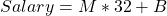We now have three unknown values. We can acquire our solution if we can determine the value of the coefficient and the intercept value. But how can we be sure that they are the best values for our data? The mathematical formulas for M and B are shown in the diagram below.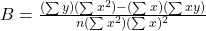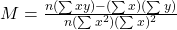Consider some data that is already plotted on the 2d graph to better comprehend. The goal now is to determine the best-fitting linear line for the provided data. However, you might have a lot of lines. So, how do we pick the best one?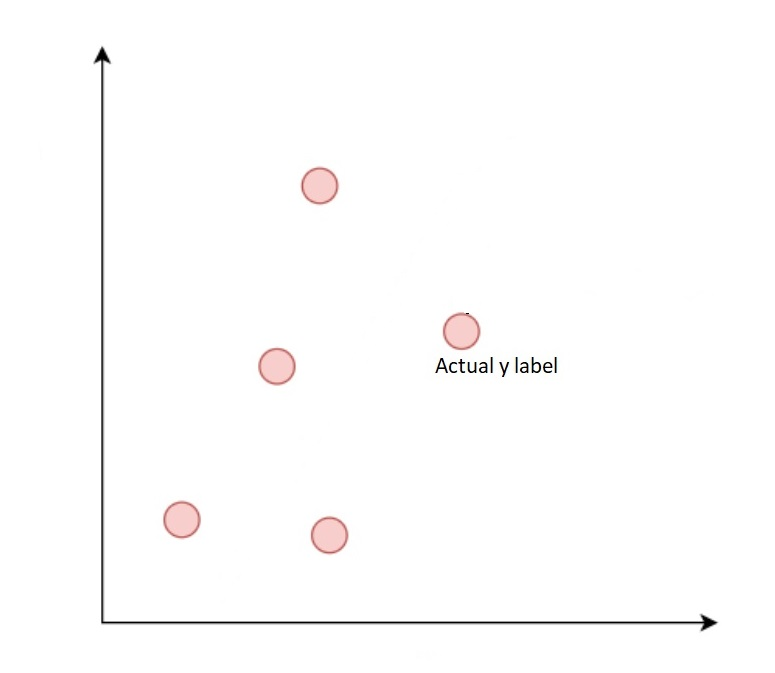Graphical representation of data

Data with random lines are shown in the diagram below.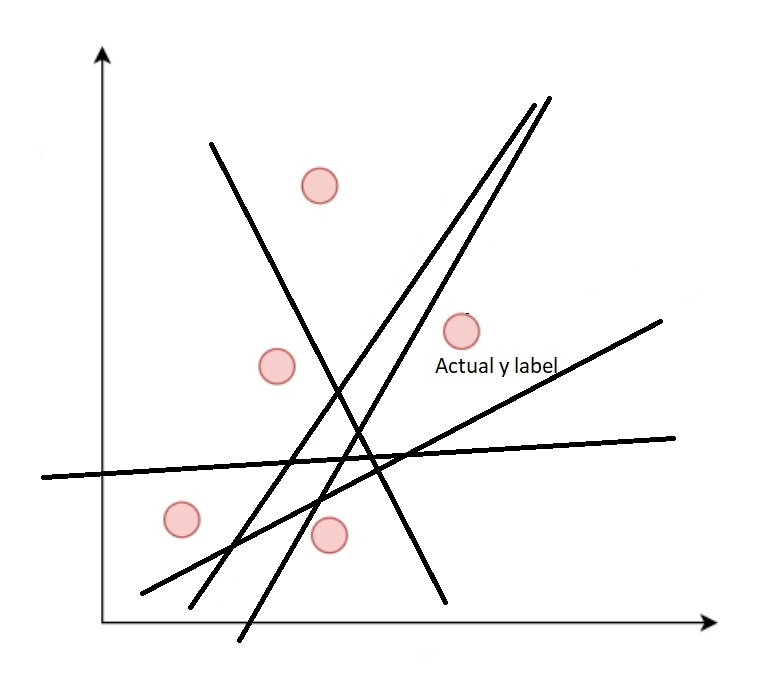Graphical representation of data with random lines

Let's pretend there's only one line. Calculate the error, add all of the errors together, then divide the total by the number of data points. The cost function, often known as the mean squared error, is the result. We may now replace y predicted with a line equation. Calculate the cost function for all random lines now. For the given data set, the line that shows the smallest cost function is the best-fitted line. The cost function for a single random line is calculated in the diagram below.Graphical representation of data with random line and cost function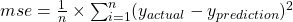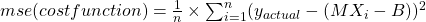However, calculating the cost function for each random line is inefficient. It is a challenging task.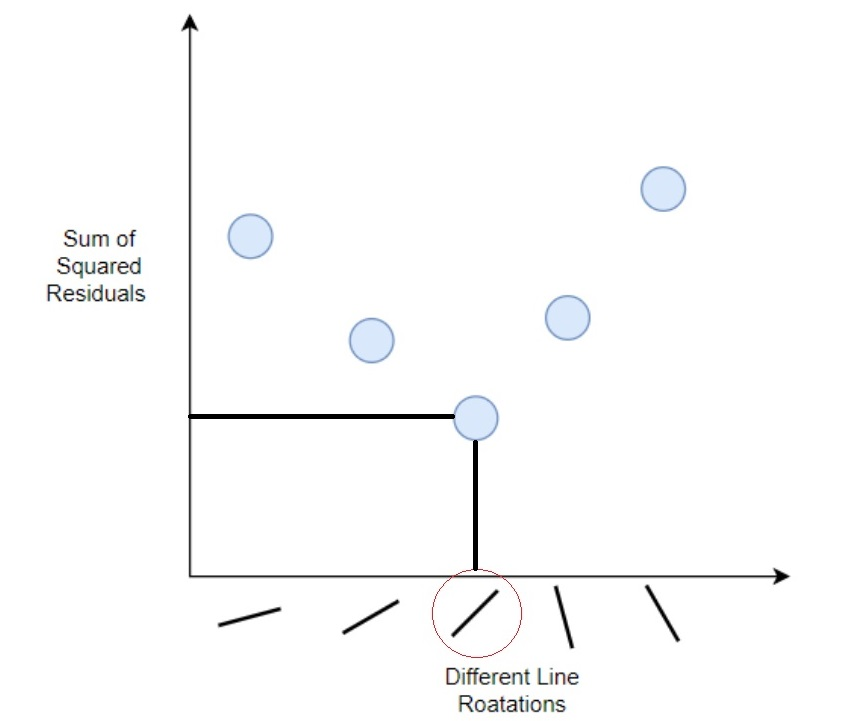The graph above can also be obtained by plotting the cost function (sum of squared residuals) against line rotation. The key to be highlighted is that the value is optimal and represents the lowest cost function. If we look at the line's rotation, we can see that this line is the greatest match for the data. But, though we covered a graphical way, getting the global lowest point of a function in the actual world is not that simple. So, what other options do we have?

Gradient descent is an algorithm that assists us in quickly determining the best fit of a line.The above graph is plotted mean squared error against M and B(C). To find the global minima, we must begin with any random value. Reduce the values of M and B by a certain amount in the next phase. Repeat these procedures until the graph's global minima are reached. We took small moves to reach the least point. But how do we go about taking those baby steps?

We can divide this three-dimensional graph into two two-dimensional graphs. Still, for the sake of simplicity, we'll analyze anyone graph.

Case1:

Now, in the below graph, we've taken fixed steps to achieve the minimum point. Still, I missed the global minimum point, and my gradient descent has never converged in this case. In gradient descent, this phenomenon is known as overshooting.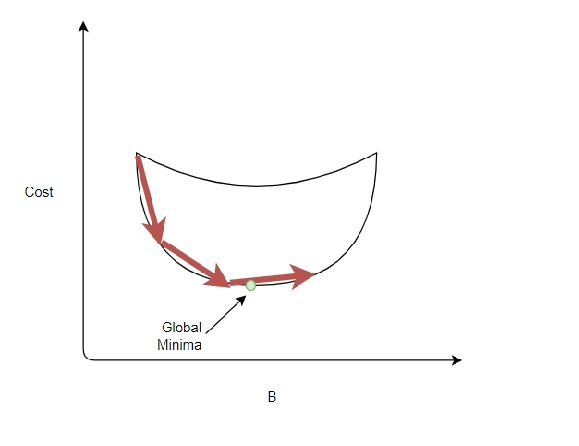Case2:

In this scenario, we're following the curve's curvature and reducing the size of the steps as we approach the global minimum point. We can have global minima if we can do anything like this.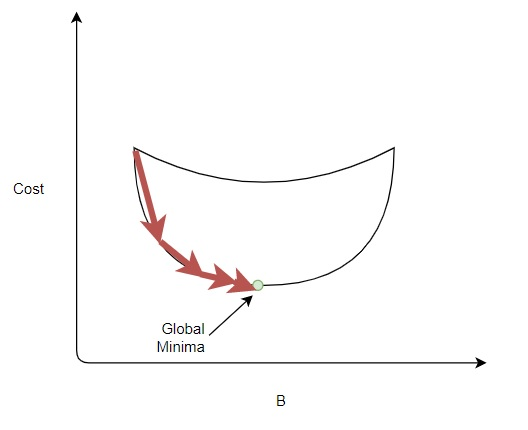Reached to global minima

We must take certain steps to implement this strategy. A graphical depiction is shown in the diagram below.

Consider one point B0; to obtain this point, we require the slope of that point. The slope at that point also indicates in which direction we must take steps and the learning rate we must consider to reach the following point. The partial derivative of B with respect to the cost function is the slope in this case.Updated Formula

To get B1, remove the partial derivative of B from Bo together with the learning rate.

The cost function equation, the partial derivative with M, and the partial derivative with B are shown in the graphic below. Then, after we obtain partial derivative, we may identify the optimal values of M and B by understanding steps, also known as a learning rate. As you can see, to calculate updated values of M and B, we must subtract the slope from old values of M and B and the learning rate.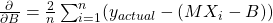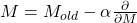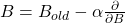Learning rate and number of iterations will be crucial factors in obtaining global minima. Let's take a coding technique to make things clearer.

## MATLAB Coding

%%%%%%%%%%%%%%%%%%%%%%%%%%%%%%%%%%%%%
% Topic : How does gradient descent work?.
% Organization : MATLAB Helper
% Website: https://MATLABHelper.com
% With the help of this script, we learn how gradient descent work internally.
%% Initial Commands
clc;
clear all;
close all;
syms x
f=x^2-3*x+2;
df=2*x-3;
fplot(f,[-10 15]);
pause(1);
hold on;
%% Random Guess
x0=12;
fx0=110;
x_value=[];
y_value=[];
for i=1:120 % Number of Iterations
x_value=[x_value x0];
y_value=[y_value fx0];
x0=x0-0.001*(2*x0-3);
fx0=x0^2-3*x0+2;
plot(x_value,y_value,'r-*','linewidth',1);
pause(0.02);
end

Our function is solely dependent on one argument in the code. For each x value, Fx0 has determined a function value. We started with 12 as a random estimate in the above manner; hence we received 110 as a function value for 12. After that, the code iterated and updated the values of x and y, where x is the value of one argument and y is the value of the function.

### MATLAB Output

When the learning rate is equal to 0.001, the path of the algorithm is shown in the diagram below.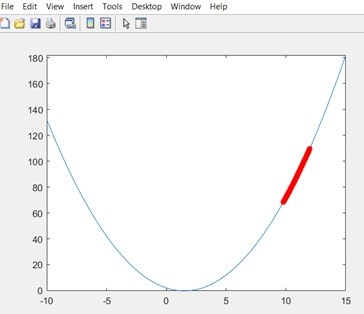Function is not diverged

The path is not converged to global minima.

When the learning rate is equal to 1, the path of the algorithm is shown in the diagram below.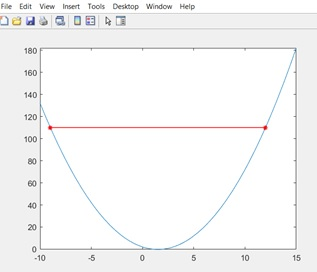Overshooting approach

The path has shown overshooting in gradient descent.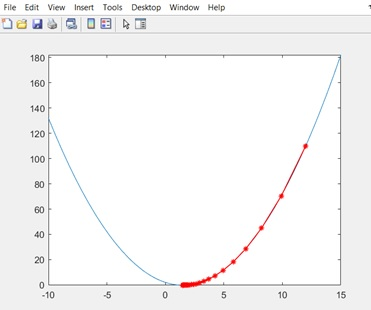Function is Converged

When the learning rate is equal to 0.1, the path of the algorithm is shown in the diagram above.

The path is converged to global minima.

## Conclusion

• Gradient Descent is an optimization approach for locating a differentiable function's local minimum. Gradient descent is a method for determining the values of a function's parameters that minimize a cost function to the greatest extent possible.
• During gradient descent, the learning rate is utilized to scale the magnitude of parameter updates. The learning rate value you choose can have two effects: 1) the speed with which the algorithm learns, and 2) whether or not the cost function is minimized.
• Machine learning and deep learning approaches are built on the foundation of the Gradient Descent method.

Ready to take your MATLAB skills to the next level? Look no further! At MATLAB Helper, we've got you covered. From free community support to expert help and training, we've got all the resources you need to become a pro in no time. If you have any questions or queries, don't hesitate to reach out to us. Simply post a comment below or send us an email at [email protected].

And don't forget to connect with us on LinkedIn, Facebook, and Subscribe to our YouTube Channel! We're always sharing helpful tips and updates, so you can stay up-to-date on everything related to MATLAB. Plus, if you spot any bugs or errors on our website, just let us know and we'll make sure to fix it ASAP.

Ready to get started? Book your expert help with Research Assistance plan today and get personalized assistance tailored to your needs. Or, if you're looking for more comprehensive training, join one of our training modules and get hands-on experience with the latest techniques and technologies. The choice is yours – start learning and growing with MATLAB Helper today!

Education is our future. MATLAB is our feature. Happy MATLABing!

Greetings from Anish !!!. I graduated from Veermata Jijabai Technological Institute in 2019 with a Master's degree in Control System. I'm obsessed with all tech-related things, and I'd like to succeed in a stimulating and challenging environment that will provide me with opportunities for advancement.

•Shylaja Vinaykumar Karatangi says:

It was nice explanation, complete understanding the concept. May I get your mail ID for my future work help. Thank you

•tanu jain says:

nice way for matlab based code for algo concept

#### MATLAB Helper ®

Use Website Chat or WhatsApp at +91-8104622179